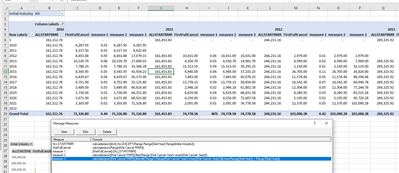cancel
Showing results for
Did you mean:Helper V

## Having trouble getting a rolling sum to work properly

I have a dataset that provides "Starting RMR" (RMR is recurring monthly revenue) by year but then also shows the first cancellation by year. So in the pivot below I have RMR by start year going across the top and then by cancel year going down the left hand column.

As you can see, I have  measure that brings back all of the started RMR by year and then also a measure that calculates the cancelled as of the year it cancelled. So I can easily show the % cancelled in any given year of a given start Year. For example, below you can see we added \$244,231 in 2012 and \$16,310 of this cancelled in 2017.

What I want to do is have this as a *Rolling* sum. What I want to show where \$16,310 is shown instead is \$16,310 plus every number before that (12,467  ,  27,808, etc. etc. etc.).

I have a date table... I just can't quite get it to work. Any ideas?

Thanks,1 ACCEPTED SOLUTIONHelper V

In the Dax formula below/second image below you'll see *a* solution. Not sure if it's the best one. But there's another weird problem. The Pivot Table doesn't sum the row grand totals of the rolling measure properly, even though everything else is summed and rolls properly within the columns. See first image below. The row grand total takes only the latest in the row into of summing it. Hmm.

``=calculate(sum([First Cancel RMR]),filter(allselected('Range'),Range[First Cancel Year]<=max([First Cancel Year]) && max(Range[StartYear]) = 'Range'[StartYear]))``5 REPLIES 5Super User

Please provide sample data in a tabular structure (not an image) or sample PBIX file & a depiction of the expected outcome (from Excel for example)

In doing so, you are also helping me. Thank you!

Proud to be a Super User!Helper V

I think I'm getting close with this... it's rolling, just rolling too much. need to add some more filter.Helper V

Attached is a link to a file with more test data. You can see what I'm after on the summary tab. I really just need a rolling figure. I can get it to work except for the rolling % piece.Helper V

This actually works (the blue highlighted) but I need to get it to work when different industries are selected..Helper V

In the Dax formula below/second image below you'll see *a* solution. Not sure if it's the best one. But there's another weird problem. The Pivot Table doesn't sum the row grand totals of the rolling measure properly, even though everything else is summed and rolls properly within the columns. See first image below. The row grand total takes only the latest in the row into of summing it. Hmm.

``=calculate(sum([First Cancel RMR]),filter(allselected('Range'),Range[First Cancel Year]<=max([First Cancel Year]) && max(Range[StartYear]) = 'Range'[StartYear]))``